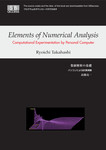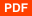# Elements of Numerical Analysis

エスアイビー・アクセス

3,300円 (3,000円+税)パソコンによる計算実験ができる数値解析の入門書。収録ソフトウェアは株式会社エスアイビー・アクセスからダウンロードできる。

### 関連書籍

• 高橋亮一『数値解析の基礎–パソコンによる計算実験』

### 内容紹介

This book intends to use a personal computer efficiently to learn elements of numerical analysis on screen, because a todayﾕs personal computer may be as powerful as a yesterdayﾕs super computer.It shows utilization of increasing power of calculation and visualization for nonlinear finite difference systems, because many practical problems are too complex to allow detailed calculation.It bridges the large gap for student between mathematical truths and related computational techniques on screen, because mathematical books may imply useful information but their descriptions are not always easy to apply directly to practical problems.It equips computational apparatus: 21 Visual Basic programs in it to explain numerical systems mathematically, to devise efficient and robust algorithms and to provide physical analogy to given numerical systems on screen, because the important computational concepts and their utilities, thereby, should be illustrated in application-oriented sense.It contains applicable engineering problems in miniature, because careful studying source code may be most efficient and easiest to understand an incorporated algorithm and to produce an own variant from the miniature.The book insists that our first interest is in physical phenomena, and in mathematics only as it relates to physics. Computational physics and engineering may be a separate discipline, distinct from experimental and from theoretical, with its own techniques, its own difficulties and its own utility.

### 書誌情報

• 著者: Ryoichi Takahashi
• 発行日: (紙書籍版発行日: 2008-11-10)
• 最終更新日: 2017-04-10
• バージョン: 1.0.0
• ページ数: 200ページ(PDF版換算)
• 対応フォーマット: PDF
• 出版社: エスアイビー・アクセス

### 著者について

#### Ryoichi TakahashiRyoichi Takahashi is a professor emeritus at Tokyo Institute of Technology. He has graduated from a nuclear engineering major, the graduate school of Tokyo Institute of Technology and received a doctor of engineering from the same university. He has worked for the research laboratory for nuclear reactors and the department of mechanical engineering of the same university as a research associate, assoc. professor, professor and professor emeritus since 1996. He was awarded a prize of Atomic Energy Society of Japan (1988). He has (co-) authored 120 publications and 4 scientific books in the fields of two-phase flow dynamics in nuclear engineering, application of lattice gas automata theory to mixture dynamics in computational physics and fuzzy control of iterative solver for nonlinear elliptic equation in numerical analysis.

### 目次

#### Chapter 1 Introduction

• 1.1 Computer as telescope
• 1.2 Four processes for numerical simulation on screen
• 1.2.1 Example of an apparatus for numerical experiment
• 1.3 Formulation / Modeling
• 1.4 Transformation / Discrete approximation
• 1.5 Computation / Algorithm
• 1.6 Computation / Coding
• 1.7 Visualization
• 1.8 Feedbacks / Validation + Verification
• 1.9 Remarks

#### Chapter 2 Partial Differential Equation and Analytical Solution — Hyperbolic, Parabolic and Elliptic Equations —

• 2.1 Type of partial differential equation
• 2.2 Type of boundary condition
• 2.3 Preliminary for analytical solution
• 2.3.1 Expansion of rectangle in terms of {sin(2m - 1) πx}
• 2.3.2 Analytical solution of heat conduction equation on screen
• 2.4 Hyperbolic PDE — Analytical solution of wave equation —
• 2.5 Parabolic PDE — Analytical solution of heat conduction equation —
• 2.6 Elliptic PDE —Analytical solution of Poisson equation —

#### Chapter 3 Finite Difference Equation and Computational Molecules— Discrete approximation and scheme —

• 3.1 Mesh system on screen
• 3.2 Difference quotient for differential operator
• 3.3 Discrete approximation for wave equation
• 3.4 Discrete approximation for advection equation
• 3.5 Discrete approximation for heat conduction equation
• 3.6 Discrete approximation for the Poisson equation
• 3.7 Nature of finite difference method comparing with FEM

#### Chapter 4 Change in Qualitative Nature of System— Initial value problem —

• 4.1 Preliminary for finite difference scheme
• 4.2 Stability of dynamic system on screen
• 4.4 von Neumann stability analysis
• 4.5 Connection between consistency and numerical instability on screen
• 4.6 Artificial viscosity
• 4.7 Ill-posed problem

#### Chapter 5 Iterative method for Elliptic Difference Equation— Boundary value problem —

• 5.1 Model question Q7
• 5.1.1 Ordering of lattice points on screen
• 5.2 Model question Q8, Q9 and Q10
• 5.3 Basic aspect of iterative methods
• 5.4 Jacobi method
• 5.5 Gauss-Seidel method
• 5.6 SOR method — Successive Over Relaxation method —
• 5.7 Analogy of iteration to solving time-dependent question
• 5.9 Basic aspects of direct Method

#### Chapter 6 Applications— Mixed natures of PDEs —

• 6.1 Laplace equation with variable coefficient — Inner and outer iteration for nonlinear system —
• 6.2 Poisson equation with variable coefficient — Inner and outer iteration for nonlinear system —
• 6.3 Soliton — Boussinesq Equation, solitary waves or balls —
• 6.4 Burgers equation — Numerical treatment of discontinuity —
• 6.5 Back step flow — Basic algorithm for mixed nature of PDEs —
• 6.6 Collision of water droplets — VOF: Algorithm for flow with free surface —
• 6.7 Blow down — Semi-implicit scheme for flow with phase change and wave propagation —

#### Index

Home 書籍一覧 Elements of Numerical Analysis